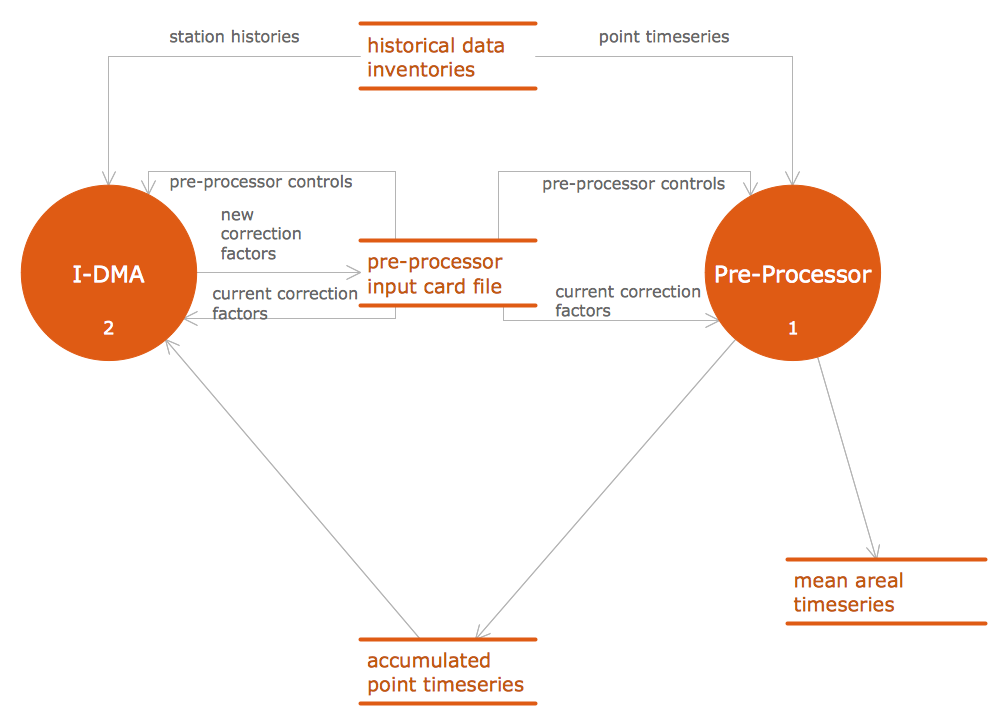This site uses cookies. By continuing to browse the ConceptDraw site you are agreeing to our Use of Site Cookies.
ConceptDraw Samples | Software development — Data flow diagrams

# Data Flow Diagrams (DFD)

Samples of data flow diagrams (DFD) are created using ConceptDraw DIAGRAM diagramming and vector drawing software enhanced with Data Flow Diagrams solution from ConceptDraw Solution Park.

ConceptDraw DIAGRAM provides export of vector graphic multipage documents into multiple file formats: vector graphics (SVG, EMF, EPS), bitmap graphics (PNG, JPEG, GIF, BMP, TIFF), web documents (HTML, PDF), PowerPoint presentations (PPT), Adobe Flash (SWF).

## Tutorials and Solutions:

Video Tutorials: ConceptDraw Solution Park

Solutions: Data Flow Diagrams for ConceptDraw DIAGRAM

## Sample 1: DFD — Model of Small Traditional Production Enterprise

Data Flow Diagrams sample: Model of small traditional production enterprise.

This example is created using ConceptDraw DIAGRAM diagramming software enhanced with Data Flow Diagrams solution from ConceptDraw Solution Park.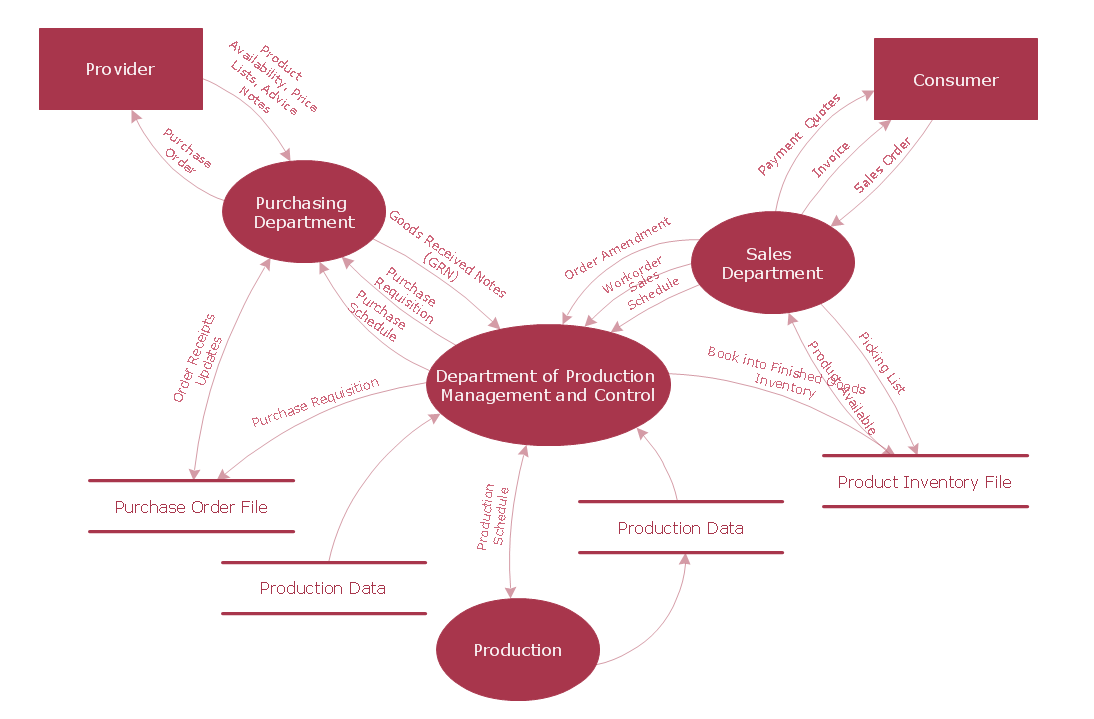## Sample 2: DFD — Process of Account Receivable

Data Flow Diagrams sample: Process of Account Receivable.

This example is created using ConceptDraw DIAGRAM diagramming software enhanced with Data Flow Diagrams solution from ConceptDraw Solution Park.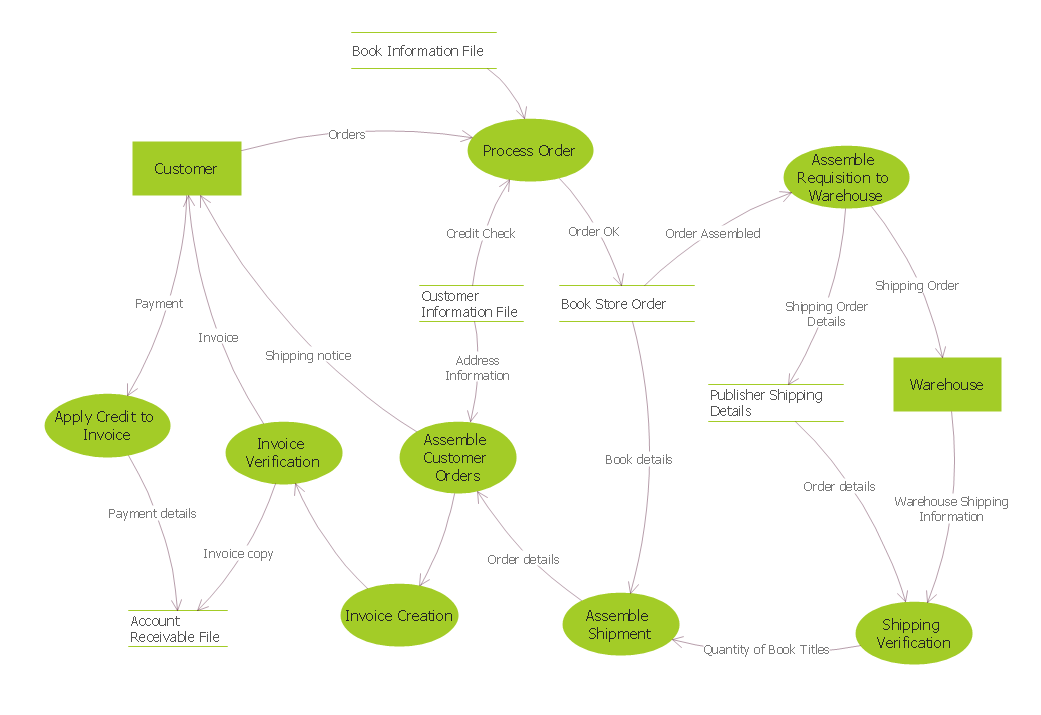## Sample 3: Data Flow Diagram (DFD)

Data Flow Diagrams sample: Data flow diagram.

This example is created using ConceptDraw DIAGRAM diagramming software enhanced with Data Flow Diagrams solution from ConceptDraw Solution Park.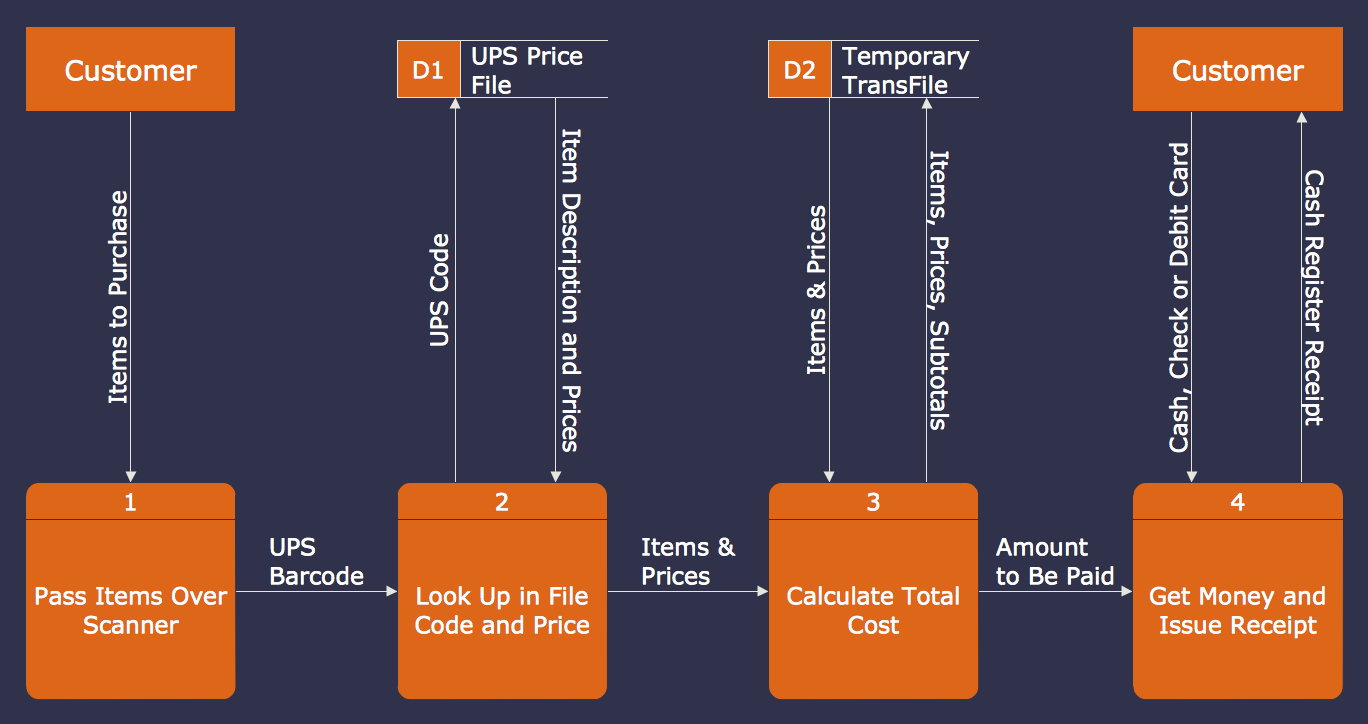## Sample 4: DFD — CERES

Data Flow Diagrams sample: CERES.

This example is created using ConceptDraw DIAGRAM diagramming software enhanced with Data Flow Diagrams solution from ConceptDraw Solution Park.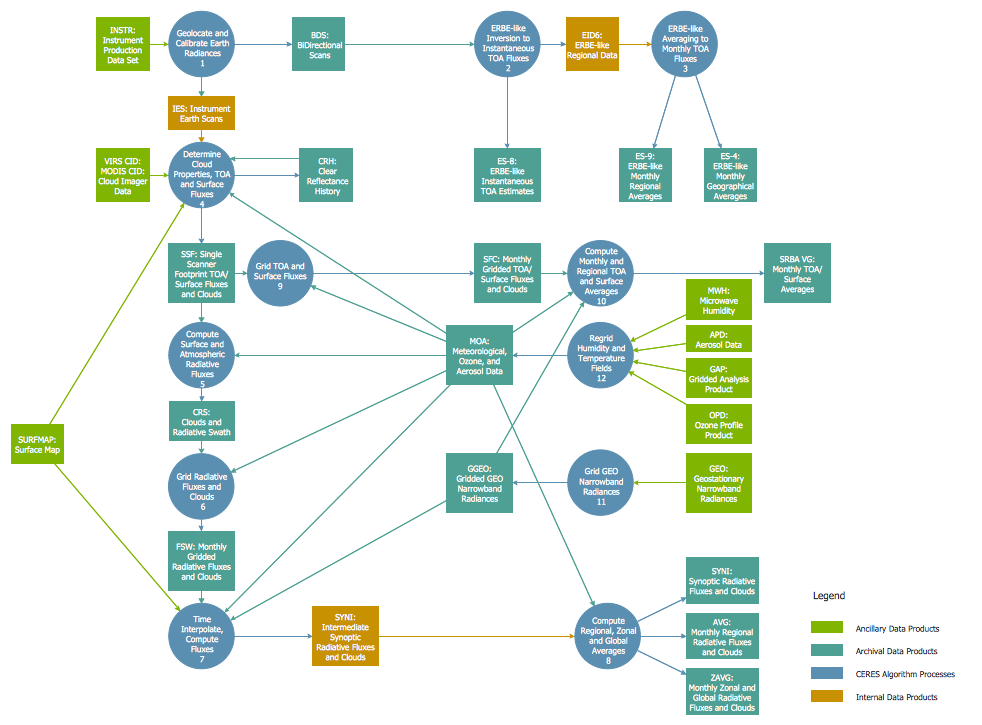## Sample 5: DFD — Coad and Yourdon Object Oriented Analysis Model

Data Flow Diagrams sample: Coad and Yourdon object oriented analysis model.

This example is created using ConceptDraw DIAGRAM diagramming software enhanced with Data Flow Diagrams solution from ConceptDraw Solution Park.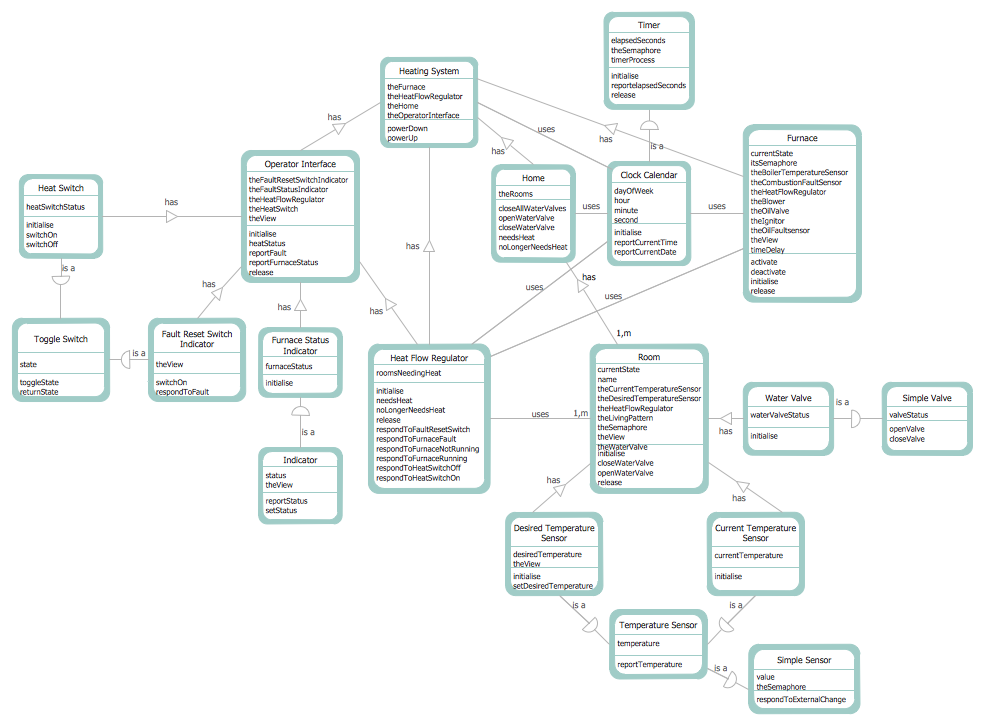## Sample 6: DFD — Last Resort Hotel Book Room Process

Data Flow Diagrams sample: Last resort hotel book room process.

This example is created using ConceptDraw DIAGRAM diagramming software enhanced with Data Flow Diagrams solution from ConceptDraw Solution Park.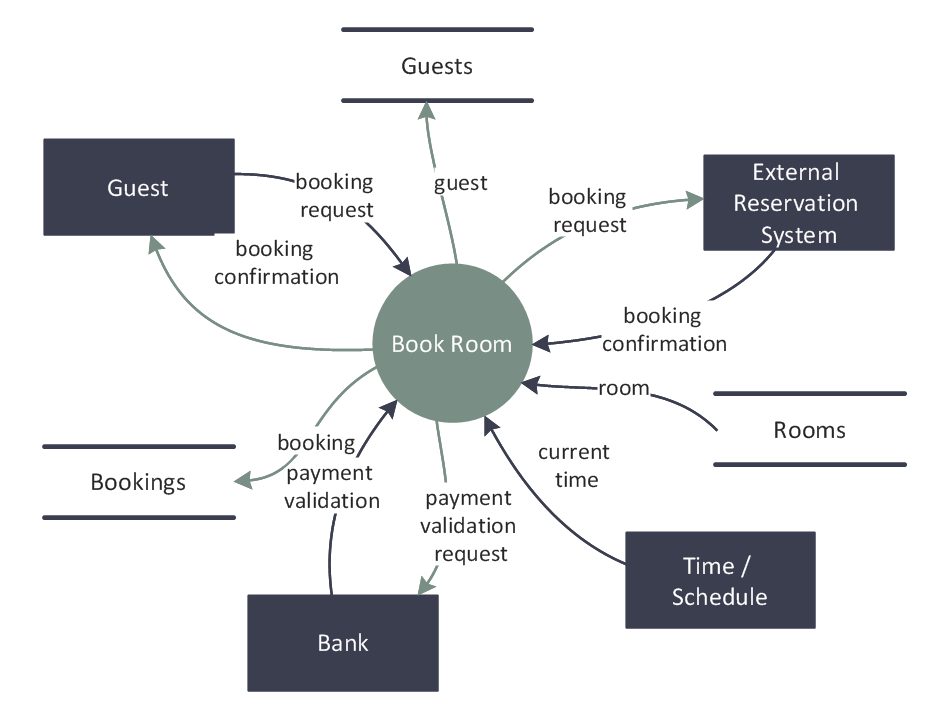## Sample 7: DFD — Interaction Between IDMA and Preprocessor

Data Flow Diagrams sample: Interaction between IDMA and preprocessor.

This example is created using ConceptDraw DIAGRAM diagramming software enhanced with Data Flow Diagrams solution from ConceptDraw Solution Park.